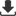ISSN 2415-1297 (Online)   ISSN 2415-1300 (Print)Volume : 27 Issue : 3 Year : 2019Current Issue Ahead of Print Most Accessed Articles Archive Online SubmissionCopyright Transfer FormLogistic Regression Analyses in Dose Response Studies [Med J Islamic World Acad Sci]
Med J Islamic World Acad Sci. 1990; 3(2): 103-106

## Logistic Regression Analyses in Dose Response Studies

From Department of Mathematics and Statistics University of Agriculture, Faisalabad, Pakistan.

In dosage response studies the two most commonly used techniques are i) Probit analysis, ii) Logit analysis. Logit analysis is easy to carry out and most practical situations are closer to logit than probit analysis. In logit analysis the function to fit is (t)= ea + at / 1 + ea+at where it is the log dose and (t) is the proportion killed. The parameters and in the function can be estimated by using following methods: Least squares, Method of moments, Weighted least squares, Maximum likelihood both the point and interval estimates for these methods are discussed by taking three practical examples. LD50 is estimated and the intervals constructed. It has been shown that method of weighted least squares gives quite good results.

Keywords: Probit analysis, logit analysis, Least squares, Method of moments, Weighted least squares, Maximum likelihood

Faqir Muhammad, Ajmal Khan, Sohail Ahmad. Logistic Regression Analyses in Dose Response Studies. Med J Islamic World Acad Sci. 1990; 3(2): 103-106

 TOOLSFull Text PDFPrintDownload citationRISEndNoteBibTexMedlarsProciteReference ManagerShare with emailShareSend email to authorSimilar articlesPubMedGoogle Scholar